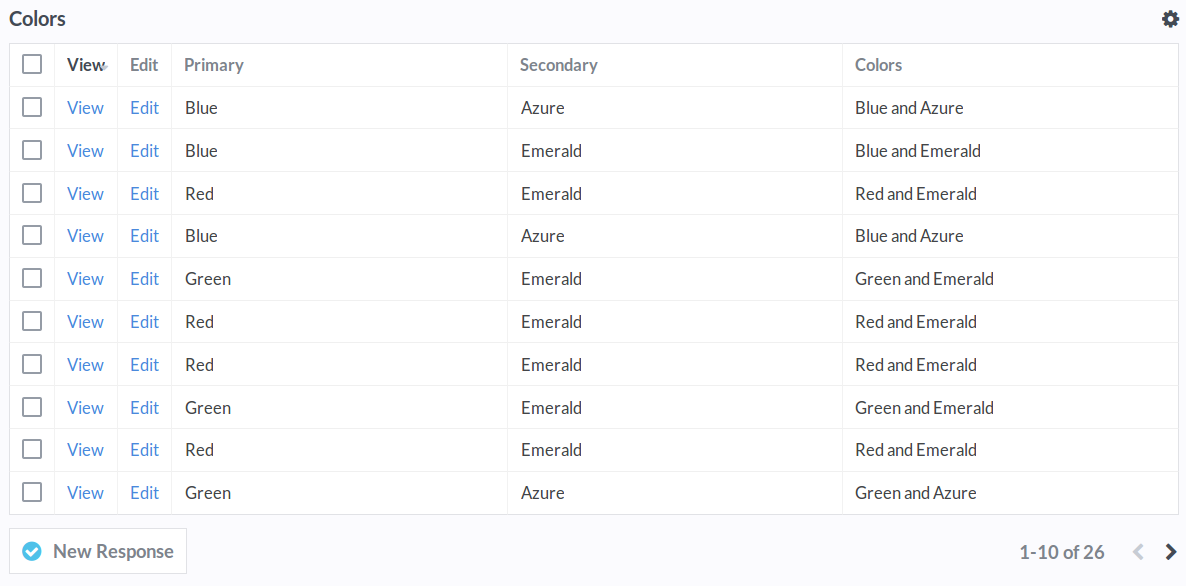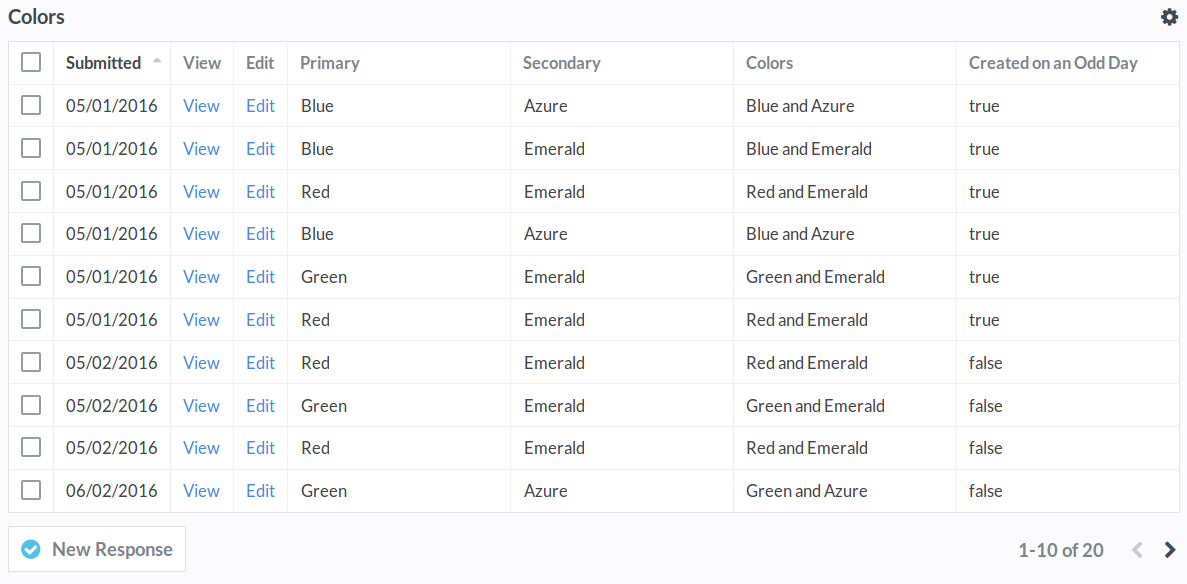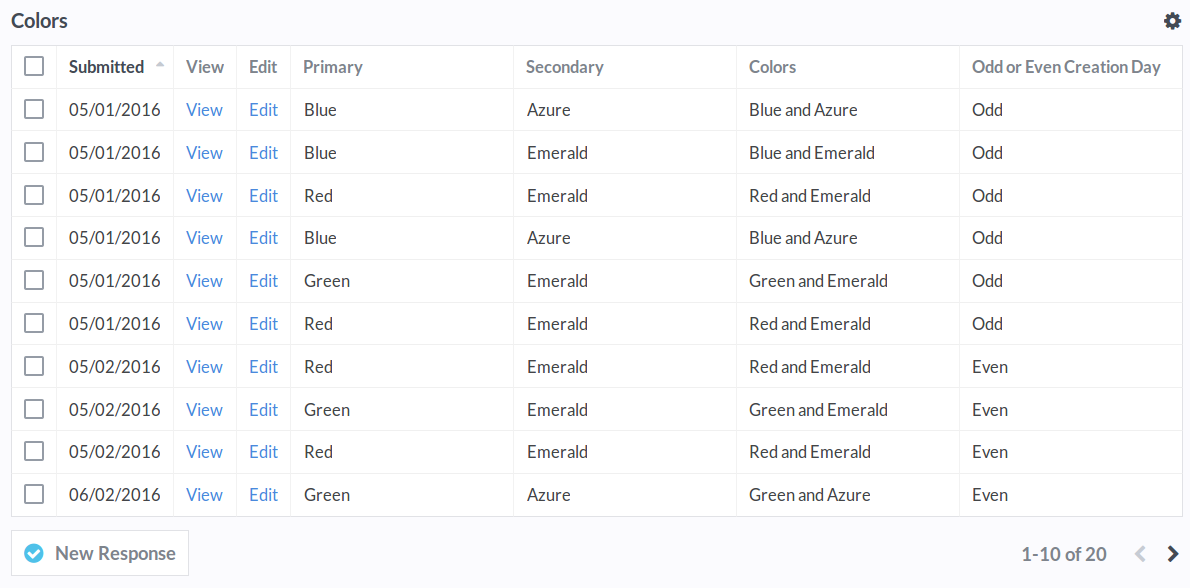# Formulas

Use Formulas to calculate values based on a submission's fields. Formulas can be used to manipulate text, calculate math values, and evaluate dates. Advanced users can check conditions and generate values.

### Getting Started

You can add formula fields from your Form Editor. Drag a Formula field to the page and open it to get started.

Formula functions should be surrounded by `{{` and `}}` - anything else will be treated as text. You'll need to use a field's variable name to get its value. By default, it's the field name lowercased with underscores instead of spaces. That means `Field Name` becomes `field_name`.

``````{{ TITLECASE(primary) }} and {{ TITLECASE(secondary) }}
``````

If the field "Primary" was submitted as "red" and the field "Secondary" was submitted as "blue", the formula above will produce:

``````Red and Blue
``````

For Multiple Choice fields, use the `ELEMENT` function to choose one of the selected options. `ELEMENT(field_name, 0)` will select the first option chosen.

### Viewing the Formula's Results

If you have a large number of submissions, formula values may not update instantly. The formula above returns the example below. Notice that the "and" was treated as text because it was outside of the `{{` and `}}` symbols.### Automatic Values

Some values, like `created`, `updated`, and `current_time`, are generated automatically.

• `created` The date and time that the submission was created. Alias of `created_at`.
• `updated` The date and time that the submission was last updated. Alias of `updated_at`.
• `current_time` The current date and time.

### Dates & Times

Use Date formulas to calculate values based on dates and times. In the example below, the formula is `IS_ODD( DAY(created_at) )`. `DAY(created_at)` selects the day of the month (1-31) from the date the submission was created on. `IS_ODD` then checks if the day's number is odd: it returns `true` if it is, and `false` if it's not.

For submissions where the day of the month is odd, like "05/01/2016", the formula returns `true`. For submissions where it's even, like "05/02/2016", the formula returns `false`.### Using Conditional Values

Using the `IF` function, you can display your own value instead of `true` or `false`. In the example below, the field "Created on an Odd Day" has been renamed to "Odd Or Even Creation Day". The formula has been changed to:

``````{{ IF( IS_ODD( DAY(created_at) ), "Odd", "Even" ) }}
``````

`IF` has multiple parameters which are separated by commas. The first parameter is the condition. If the condition is `true`, it will output the second value "Odd". If the condition is `false`, it'll output the third value "Even".### Complex Conditions

The second and third parameters of the `IF` function can be functions themselves. For example, the formula below will return either "ODD" or "EVEN".

``````{{ IF( IS_ODD( DAY(created_at) ), UPPERCASE("Odd"), UPPERCASE("Even") ) }}
``````

The parameters can even be additional `IF` functions to create more complex conditions.

``````{{
IF(
DAY(created_at) <= 14,
IF(
DAY(created_at) <= 7,
"Created in Week 1",
"Created in Week 2"
),
IF(
DAY(created_at) <= 21,
"Created in Week 3",
"Created in Week 4"
)
)
}}
``````

If the day is less than or equal to 14, the first `IF` parameter will be run. If not, the second `IF` parameter is run.

• `IF` the day is less than or equal to `14`
• `AND` the day is less than or equal to `7`
• Return `"Created in Week 1"`
• `AND` the day is `NOT` less than or equal to `7`
• Return `"Created in Week 2"`
• `IF` the day is NOT less than or equal to `14`
• `AND` the day is less than or equal to `21`
• Return `"Created in Week 3"`
• `AND` the day is `NOT` less than or equal to `21`
• Return `"Created in Week 4"`

### Notes

Formulas should be surrounded by `{{` and `}}`. Anything else will appear as text.

``````// Returns "Hello, John", if name is "john".
Hello, {{ TITLECASE(name) }}
``````

Multiple Choice fields return an array of values. To use Text functions like `TITLECASE`, you'll need to pick one of the elements. Elements start at 0, not 1, so to get the first value you will need to use `ELEMENT(primary, 0)`.

``````// Returns "Red" if "Red", "Green", and "Blue" are selected for the field "Primary".
{{ ELEMENT(primary, 0) }}

// Returns "Red". If you don't use a second parameter, it will default to 0.
{{ ELEMENT(primary) }}

// Returns "Green"
{{ ELEMENT(primary, 1) }}
``````

Function names are case-insensitive and can have spaces inside the parentheses.

``````// Returns "HELLO!"
{{ uppercase( "hello!" ) }}

// Returns "Odd" if the field "Number" is odd, and "Even" if it is not.
{{ IF ( IS_ODD( number ), "Odd", "Even" ) }}
``````

Formulas are calculated in the order they appear in the Form Editor. Formulas that come after another formula can reference its value like any other field.

``````// Returns "BLUE AND AZURE" if the "Colors" formula's value is "Blue and Azure".
{{ UPPERCASE(colors) }}
``````